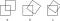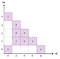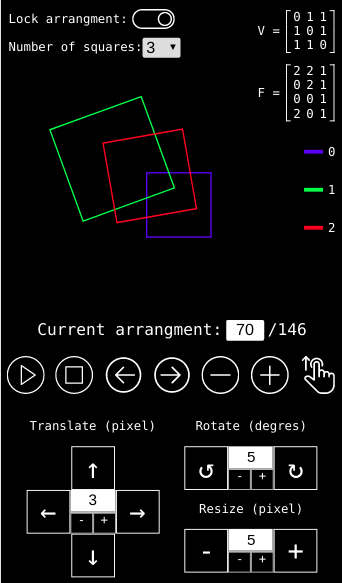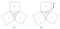# In how many ways can squares overlap?

Have you ever wondered in how many ways we can overlap 2 squares? Probably not, this is not the kind of questions we ask ourselves when shaving in the morning.

Initially I was looking for a problem where I can use my computer to do a bit of experimental mathematics. As I’m not a mathematician, I found this problem interesting because it didn’t seem to require advanced knowledge in mathematics (at least no more than a bit of analytic geometry).

Surprisingly, after many searches on Google I found nothing on this subject except a similar problem for circles on the Numberphile YouTube channel. Assuming I was walking on an unexplored territory, I decided to try to answer this question for 2 squares, 3 squares and so one… exciting !

First I had to define some reasonable rules of construction:

• 2 arrangements of n squares are considered the same if one can be continuously changed to the other without any vertex passing through an edge.
• The squares can be rescaled, rotated or translated but they must keep their square shape and be contained in a finite space, they cannot be reduced to a point.
• A vertex cannot be superimposed with an edge or with another vertex.
• Turning the whole configuration over is allowed (mirror image) and will not create a new arrangement.
• The squares are drawn in an affine plane.Figure 1. (a) and (b) represent the same arrangement because we can pass from (a) to (b) by translating and rotating the squares without having to let any vertex passing through an edge. However (b) and (c) are different because we are forced to pass one vertex through an edge in order to make the transition.

My initial idea was that calculating the exact number of arrangements possible for n squares with a brute-force algorithm could perhaps help me to find a pattern and lead me to the Holy Grail: a formula expressing Card(En) explicitly for any value of n !

But this problem, which may seem simple at first glance, is actually much more difficult than I imagined !

In this post I will use this notation:

• En for the set of arrangements formed by n squares
• En(k) for a particular arrangement k in En
• Card(En) for the number of elements of the set En

For n=1 obviously we find just one arrangement. But what happened for the big numbers ? We can guess the explosive character of Card(En), it’ll be impossible to define and draw all the arrangements when n becomes too big. For example with only 60 squares we can show that the number of possible arrangements far exceeds the estimated number of atoms in the observable universe !

It reminds me of a good quote from my math teacher Jacques Malet in 1997:

Some infinities are more muscular than others.

# For n = 2

When I first tried to draw squares on a paper for n=2, I found 10 solutions. Proud of my result I decide to ask my computer to confirm it, a simple routine operation, I thought. Here are the result…

As you can see we find 12 arrangements. Let’s be honest, it’s easy to miss one or two arrangements without the help of a computer (especially for the number 11 and 12) ! According to the rules we defined these twelve arrangements are different and we can mentally verify the impossibility to go from one to another without forcing a vertex to pass through an edge.

Here a question comes to mind: If we forced the transition from an arrangement to another by passing one vertex through an edge at a time, what would be the different paths possible between our twelve arrangements?

By performing this exercise mentally for each pair of arrangements, we can draw the graph below (where each node represents an arrangement with his number):

It is a connex graph with 3 leaves and many cycles. It is interesting to note the symmetry with respect to the vertical axis materialized by the arrangements [(7), (12), (4), (5)].

All couple of symmetric arrangements have in common the number of vertices captured (while the nature of the intersections between edges is different):

For (1) and (10): 0 vertex captured.

For (2) and (9) : 1 vertex captured by a square, 0 by the other.

For (6) and (11): 1 vertex captured by a square, 1 by the other.

For (3) and (8) : 2 vertex captured by a square, 0 by the other.

The leaves are the arrangements (1), (5) and (10) with these particularities:

(1): no vertices captured and no edge intersection

(5): no edge intersection and maximum vertices captured (4)

(10): maximum edge intersection (8) and minimum vertices captured (0)Figure 4. Distribution of the number of arrangements found based on the number of intersections (ni) and the number of vertices captured (nc).

We can show that nc cannot be an odd number and that ni and nc are linked by the relationship: nc ≤ 4 − ni / 2

# For n = 3

Card(E3) > 4618

This result could be refined as I have not yet been able to make my algorithm converge because of the limited capacity of my personal computer… But I believe I’m not far from the exact value.

Some of the arrangements I found can be seen here: http://felixdebon.com/overlappingsquaresFigure 5. This tool displays the arrangement for n = 2 and n = 3. We can move the squares (translation, rotation and rescale) and thus build new arrangements. The corresponding matrices V and F are also calculated and displayed for each arrangement.

Initially I developed this tool just to help me in the resolution of this problem and ultimately I thought it would be interesting to share it. This tool was also extremely useful to test the validity of my code.

# Calculation method

“If you want to count sheep count the legs and divide by 4.” — Jacques Malet

Unfortunately we do not count arrangements like we count sheep. Understanding of how geometric objects can be represented algebraically is crucial in the resolution of this problem. In order to count my arrangements I tried to establish an bijection between the geometric objects and their algebraic representations. Anytime I’ll generate an arrangements (with a brute force algorithm) I’ll calculate its algebraic representations and check if it’s a new one or not.

Basically my algebraic representation (for n=2) is made of 2 matrices:

• V(i, j) = number of vertices from the square i included in the square j

V(i, i)=0 ∀ i because a square cannot contain its own vertices.

• F(i, j)= number of intersections found on the edge j of the square i

On a given square we count the number of intersection found on every edge by turning clockwise around the square (the first edge can be chosen randomly).

I give 2 examples below:

Note that the operations listed below will not define a new arrangement:

• Columns permutations: As the square are chosen in a random order, as long as we permute the same column in V and F, we will not define a new arrangement.
• Circular permutations on a column of F: because the first edge is chosen randomly before we start to go through each edge clockwise.
• Mirror image: as defined in the construction rules an arrangement is invariant by mirror image. We can define the mirror image transformation by: F(i, j) → F( 4-1-i, j)

I found that V and F are enough to describe unambiguously all the E2 arrangements.

Unfortunately we can prove that it doesn’t work for E3:

Notation: I will use round brackets for squares and square brackets for sub-polygons.

Let’s consider the arrangement E2(9) with the square (0) and (1). E2(9) contains 8 regions [a], [b], [c], [d], [e], [f], [g], [h]. Let’s try to draw a third square (3) such that all its vertices are included in one of the regions and only one.

As we assume that (3) doesn’t have any intersection point with (0) or (1), the F matrix is invariant:

The V matrix however can have 4 values depending on where we place the third square:

While (V, F) is the same by inserting a square in [a] or [c] we can see that the arrangements created are different because the square (3) would not be surrounded by the same polygon in [a] and [c] ([a] is a 5 vertices region while [c] has only 3 vertices). There is no way to pass from [a] to [c] with a continuous transformation because such a transformation cannot change the number of vertices of a polygon.

• [e] and [c] are equivalent because [e] is transformed into [c] by a mirror image.
• [a] and [c] are not equivalent because [a] would not be transformed into [c] by a mirror image.

Conclusion: we found 2 different E3 arrangements with the same matrices V and F which proves that V and F are not sufficient to describe entirely E3.

For n=4 things are even worse, we can rearrange the first 3 squares to enclose the fourth. The 3 squares create an emerging polygon (the red triangle in the figure below). In (a) while the square (4) is not contained individually by the other squares it is contained by the emerging polygon they create.Figure 6. An example of emerging polygon created by a cycle of square.

Passing the square (4) through an edge or breaking the cage will violate the rules we defined. Therefore the 2 arrangements (a) and (b) are strictly different. The matrix identification method built for E2 doesn’t work anymore with such exotic arrangement because the pair (V, F) remains the same as the square (4) is either inside or outside the cage.

These examples suggest that we should take into consideration more precisely where the square’s vertices are located. For this reason I introduced a new matrix.

S(i, j) = index of the sub-polygon (in the arrangement formed by the 2 other squares) in which the vertex j of square i is included

This matrix takes into account the sub-polygons created by the overlapping squares and a certain number of symmetry criterias in order to avoid counting the same arrangement several time. Given the square (i) in an E3 arrangement we must find the E2 arrangement formed by the 2 other squares and check in which sub-polygons the vertex of (i) are located. We repeat this operation for the 2 other squares to completely write the S matrix.

# Sub-polygons (used for S matrix)

Each E2 arrangement can be split in different regions or sub-polygons. I assigned them a number. I also determined the symmetrical regions in each E2 arrangement. By convention I give the number  to the “outside area”. We consider that a point is in  if and only if it is neither in (0) nor in (1) (or in none of the sub-polygons). In E2(11) for example we find 5 sub-polygons.  and  are symmetrical. Same thing for  and .

For a given E2 arrangement the sub polygons are invariant in kind and in number (their number of vertices will not change if we apply a continuous transformation to the arrangement). This property is not true anymore in E3 but we just need it to be true for n=2.

Example 1: We compare the E3 arrangements form by moving (2) in 2 different positions as shown on the diagram below.

V and F matrices are identical for both positions of square (2):

(0) + (1) form E2(9), 7 sub-polygons, symmetries: (, ), (, )

(0) + (2) form E2(4), 3 sub-polygons, symmetries: ∅

(1) + (2) form E2(6), 3 sub-polygons, symmetries: (, )

Let’s calculate the S matrix in both cases (square (2) on the left and on the right):

The sub-polygons  and  are symmetrical in E2(9) thus we can replace 3 by 5 in the third column of SRight which gives us

Let’s apply the mirror image transformation on it: S(i, j) → S( p-1-i, j)

Finally with just one circular permutation on column 1 and 2 we end up with the same matrix:

In this example V, F, S is invariant for the 2 arrangements. The S matrix saves the day !

Example 1: Again we compare the two E3 arrangements found by moving square (2) in 2 different positions as shown on the diagram below.

Once again S matrix seams to work pretty well !

But how to recognize the different sub-polygons in an E2 arrangement ?

I give here an example for the arrangement E2(11) but the method is similar for the others E2 arrangements. Firstly we calculate explicitly all the sub-polygons (with a polygon-clipping algorithm). For every sub-polygon we will provide an ordered list of coordinates.

 is the unique 6 vertex sub-polygon.

 and  are the 5 vertex symmetrical sub-polygons (can choose randomly who is ).

 is the unique triangle having a vertex in common with .

 is the triangle having a vertex in common with  (or more simply the last sub-polygon remaining)

# Equivalent forms (V, F, S) for N=3

In a computer simulation where we perform numerous rotations and translations on the squares, the index order for a given arrangement will change all the time. Therefore we must be able to recognize the matrices corresponding to the same arrangement. I will not give more details here but basically we can re-write the matrices (V, F, S) in 2²⁷ different ways if we take into account :

• The permutation of square index
• The circular permutations in the column of F and S
• The effect of the mirror image

With this approach, two arrangements are considered the same if their matrix (V, F, S) are in the same group of equivalent forms as described above.

I didn’t prove yet that my model make it possible to establish a bijection between the arrangements and the matrices for n=3 but I’m pretty sure it can be used to calculate a good lower bound.

# Brute force algorithm

Basically I place a fixed square in the center of the simulation zone and I scan the zone with 2 other squares by repeatedly:

• Moving their centers through a grid of points.
• Changing their size (rescaling).
• Changing their orientation angle (from 0 to 90 degrees).

Anytime I generate an arrangements I calculate its algebraic representations and check if it’s a new one or not.

I used different optimization strategies to make my code run faster. For example when I generate an arrangement I don’t try to compare it frontally with all the arrangements already found.

Instead of that I store each arrangement in a particular category. When a new arrangement is generated I search its equivalent only inside this category as I’m sure that if this arrangement already exist I will find it there. The category of an arrangement is defined by 3 integers nV, nF and nS used (after concatenation) as the entry of an array.

nV is calculated as following:

I first sum the values of each column of V and store it in an array sV

I sort the array

and then I calculate nV this way

I use the same method for nF and nS.

The string key will define a category.

To compare 2 arrangements a and b (only inside a given category) I first compare their V matrices (taking into account index permutations).

If Va ≠ Vb, the arrangements are different and I stop here, otherwise I compare Fa and Fb (taking into account index permutations, circular permutations on columns and mirror image).

Again if Fa ≠ Fb the arrangements are different and I stop here, otherwise I compare Sa and Sb (taking into account index permutations, circular permutations on columns and mirror image).

Although this algorithm does not constitute in any case a demonstration, it is reasonable to think that it should converge toward the maximum arrangement possible if the translation step, the angle step and the size step are chosen small enough.

# Conclusion

We can sometimes find a short cut allowing to solve a problem more quickly. I suspect that it might not be the case for this problem (no computational reducibility). But anyway I had a lot of fun working on it and writing my brute force algorithm in pure JavaScript. At the moment I’m writing this conclusion I’m still trying to push my calculation further to get a more accurate value of Card(E3). I will try to update this post soon. Feel free to leave a comment if you find a mistake or if you have a better idea on how to solve this problem !

Written by

## More From Medium

### Growth when Innovation is Combinatorial

#### Also tagged Programming

Welcome to a place where words matter. On Medium, smart voices and original ideas take center stage - with no ads in sight. Watch
Follow all the topics you care about, and we’ll deliver the best stories for you to your homepage and inbox. Explore
Get unlimited access to the best stories on Medium — and support writers while you’re at it. Just \$5/month. Upgrade